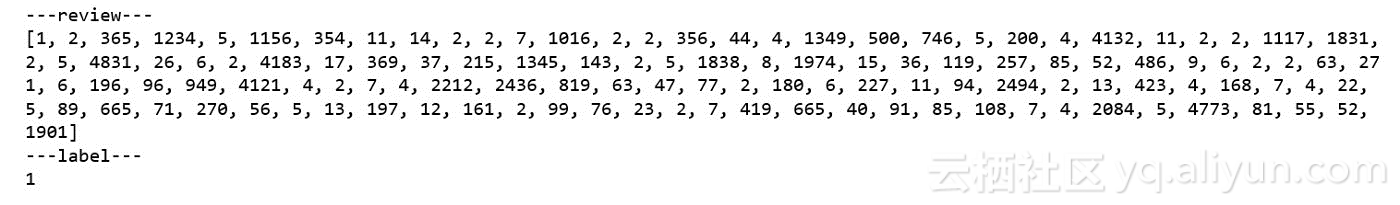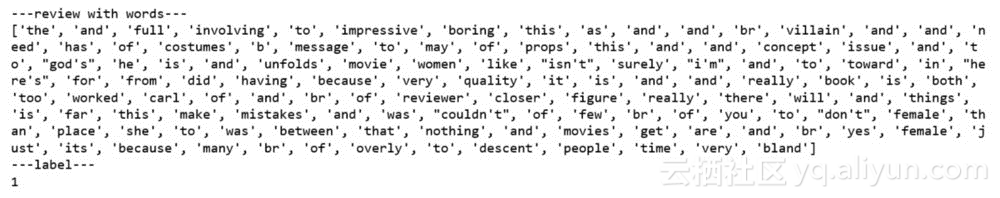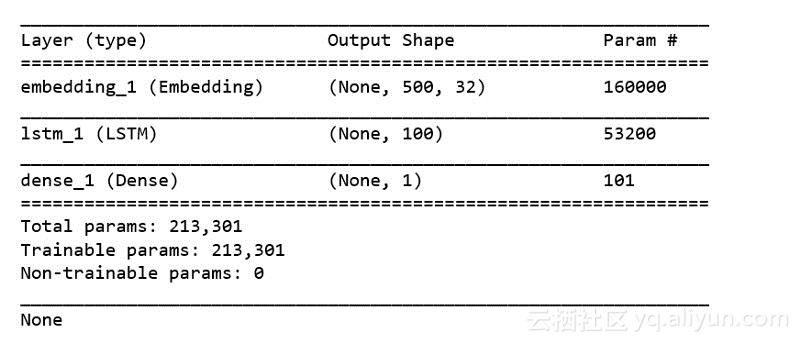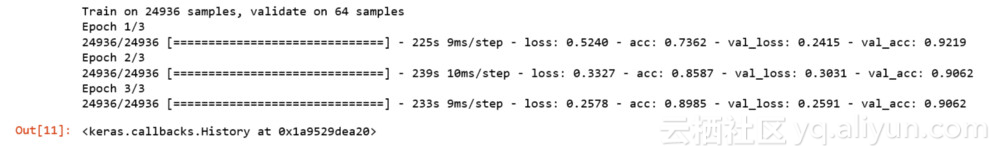0
0
01. 云栖社区>
2. 专知>
3. 博客>
4. 正文

手把手教你用RNN做情感分析—初学者指南（附代码）

A Beginner’s Guide on Sentiment Analysis with RNN

https://arxiv.org/ftp/arxiv/papers/1801/1801.07883.pdf

from keras.datasets import imdb

vocabulary_size = 5000
(X_train, y_train), (X_test, y_test) = imdb.load_data(num_words =
vocabulary_size)
print('Loaded dataset with {} training samples, {} test samples'.
format(len(X_train), len(X_test)))

print('---review---')
print(X_train)
print('---label---')
print(y_train)word2id = imdb.get_word_index()
id2word = {i: word for word, i in word2id.items()}
print('---review with words---')
print([id2word.get(i, ' ') for i in X_train])
print('---label---')
print(y_train)print('Maximumreview length: {}'.format(
len(max((X_train+ X_test), key=len))))

print('Minimum review length: {}'.format(
len(min((X_test + X_test), key=len))))

from keras.preprocessing import sequence
max_words = 500
X_train = sequence.pad_sequences(X_train, maxlen=max_words)
X_test = sequence.pad_sequences(X_test, maxlen=max_words)

from keras import Sequential
from keras.layers import Embedding, LSTM, Dense, Dropout
embedding_size=32
model=Sequential()
input_length=max_words))
print(model.summary())model.compile(loss='binary_crossentropy',
metrics=['accuracy'])

batch_size = 64
num_epochs = 3
X_valid, y_valid = X_train[:batch_size], y_train[:batch_size]
X_train2, y_train2 = X_train[batch_size:], y_train[batch_size:]
model.fit(X_train2, y_train2, validation_data=(X_valid, y_valid),
batch_size=batch_size, epochs=num_epochs)scores将会对应于评价指标 metrics=[‘accuracy’]。

scores =model.evaluate(X_test, y_test, verbose=0)
print('Testaccuracy:', scores)

Test accuracy: 0.86964

+ 关注

corcosa 14780人浏览